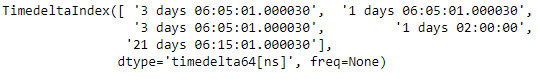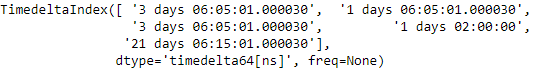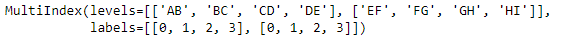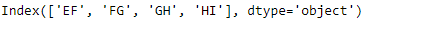Related Articles
Python | Pandas TimedeltaIndex.get_level_values
• Last Updated : 28 Dec, 2018

Python is a great language for doing data analysis, primarily because of the fantastic ecosystem of data-centric python packages. Pandas is one of those packages and makes importing and analyzing data much easier.

Pandas` TimedeltaIndex.get_level_values()` function return an Index of values for requested level, equal to the length of the index.

Syntax : TimedeltaIndex.get_level_values(level)

Parameters :
level : level is either the integer position of the level in the MultiIndex, or the name of the level.

Return : self, as there is only one level in the Index.

Example #1: Use `TimedeltaIndex.get_level_values()` function to find all the values present in the 0th level of the given TimedeltaIndex object.

 `# importing pandas as pd``import` `pandas as pd`` ` `# Create the TimedeltaIndex object``tidx ``=` `pd.TimedeltaIndex(data ``=``[``'3 days 06:05:01.000030'``, ``'1 days 06:05:01.000030'``, ``                                ``'3 days 06:05:01.000030'``, ``'1 days 02:00:00'``, ``                                                 ``'21 days 06:15:01.000030'``])`` ` `# Print the TimedeltaIndex object``print``(tidx)`

Output :Now we will use the `TimedeltaIndex.get_level_values()` function to find all the values in 0th level

 `# print values in 0th level``tidx.get_level_values(``0``)`

Output :As we can see in the output, the `TimedeltaIndex.get_level_values()` function has returned all the values present in the 0th level of tidx object. This object has only one level.

Example #2: Use `MultiIndex.get_level_values()` function to find all the values present in the 1st level of the given MultiIndex object.

 `# importing pandas as pd``import` `pandas as pd`` ` `# Create the MultiIndex object``midx ``=` `pd.MultiIndex.from_arrays(([``'AB'``, ``'BC'``, ``'CD'``, ``'DE'``], ``                                  ``[``'EF'``, ``'FG'``, ``'GH'``, ``'HI'``]))`` ` `# Print the MultiIndex object``print``(midx)`

Output :Now we will use the `MultiIndex.get_level_values()` function to find all values in the 1st level

 `# print values in 1st level``midx.get_level_values(``1``)`

Output :As we can see in the output, the `MultiIndex.get_level_values()` function has returned all the values present in the 1st level of midx object.

Attention geek! Strengthen your foundations with the Python Programming Foundation Course and learn the basics.

To begin with, your interview preparations Enhance your Data Structures concepts with the Python DS Course.

My Personal Notes arrow_drop_up